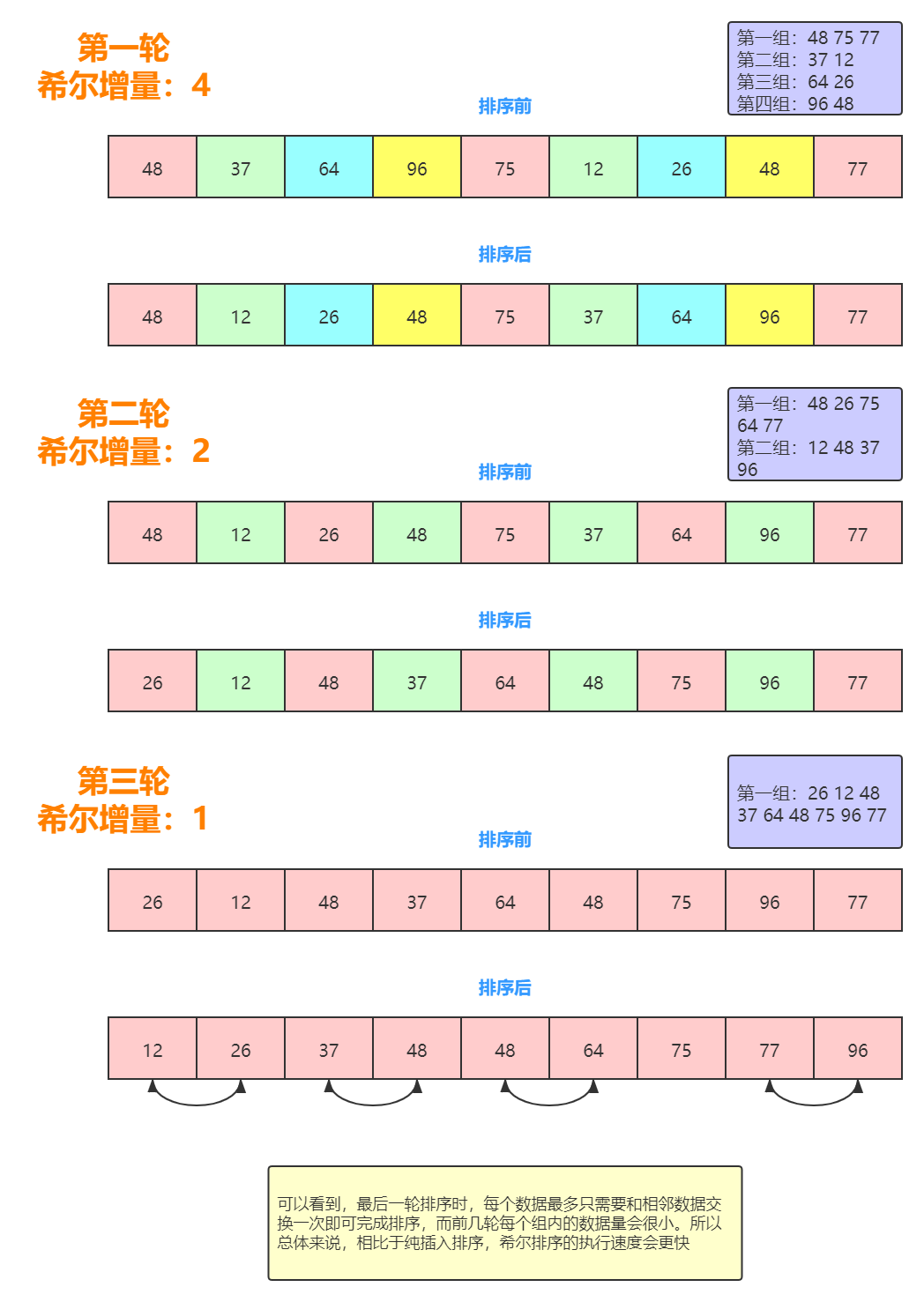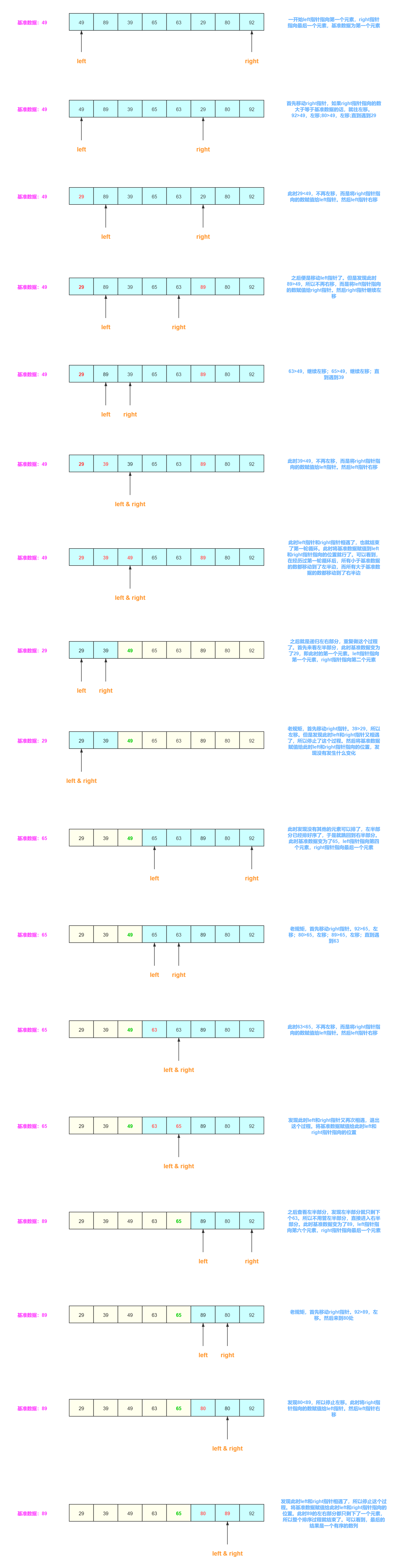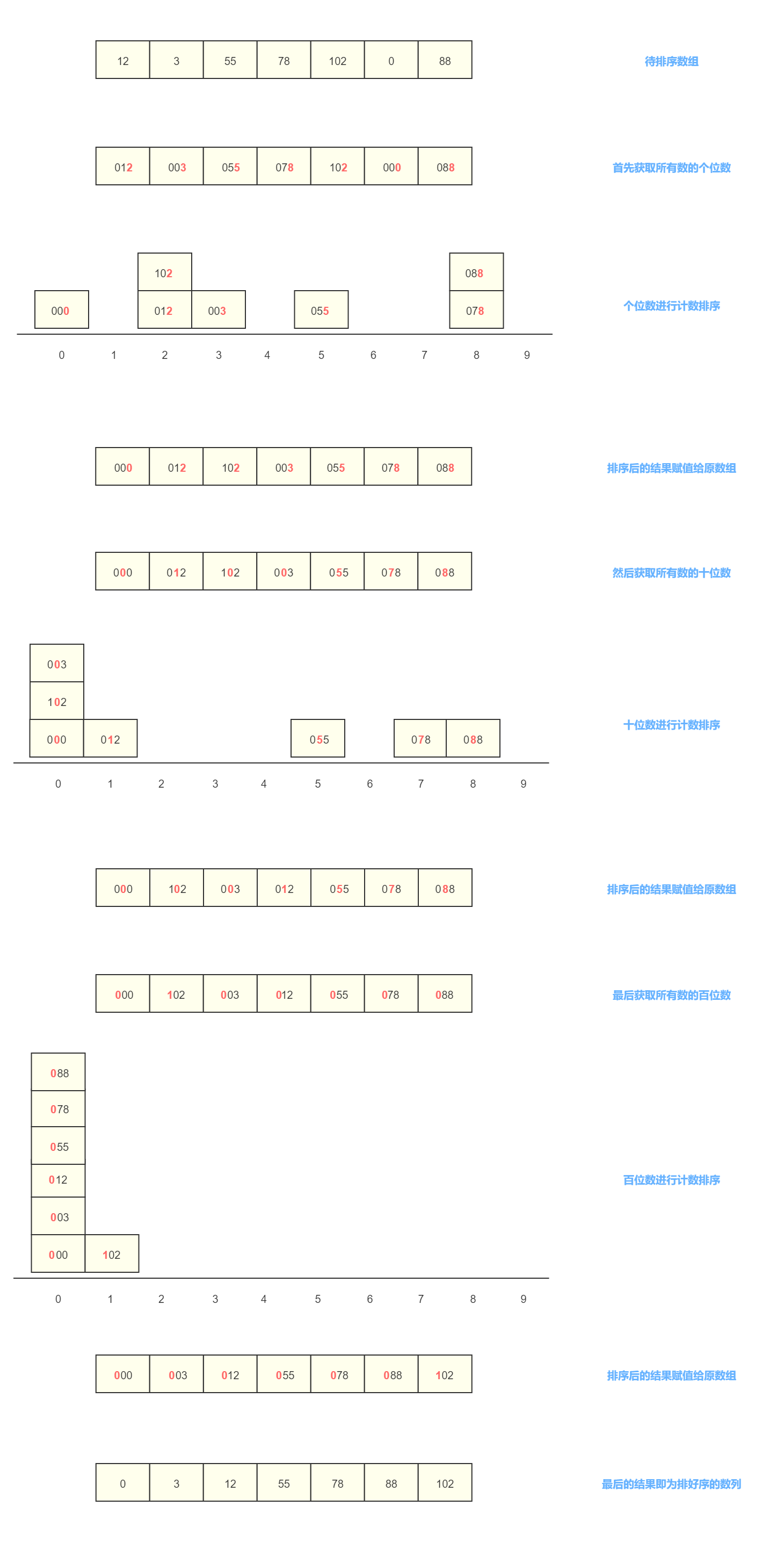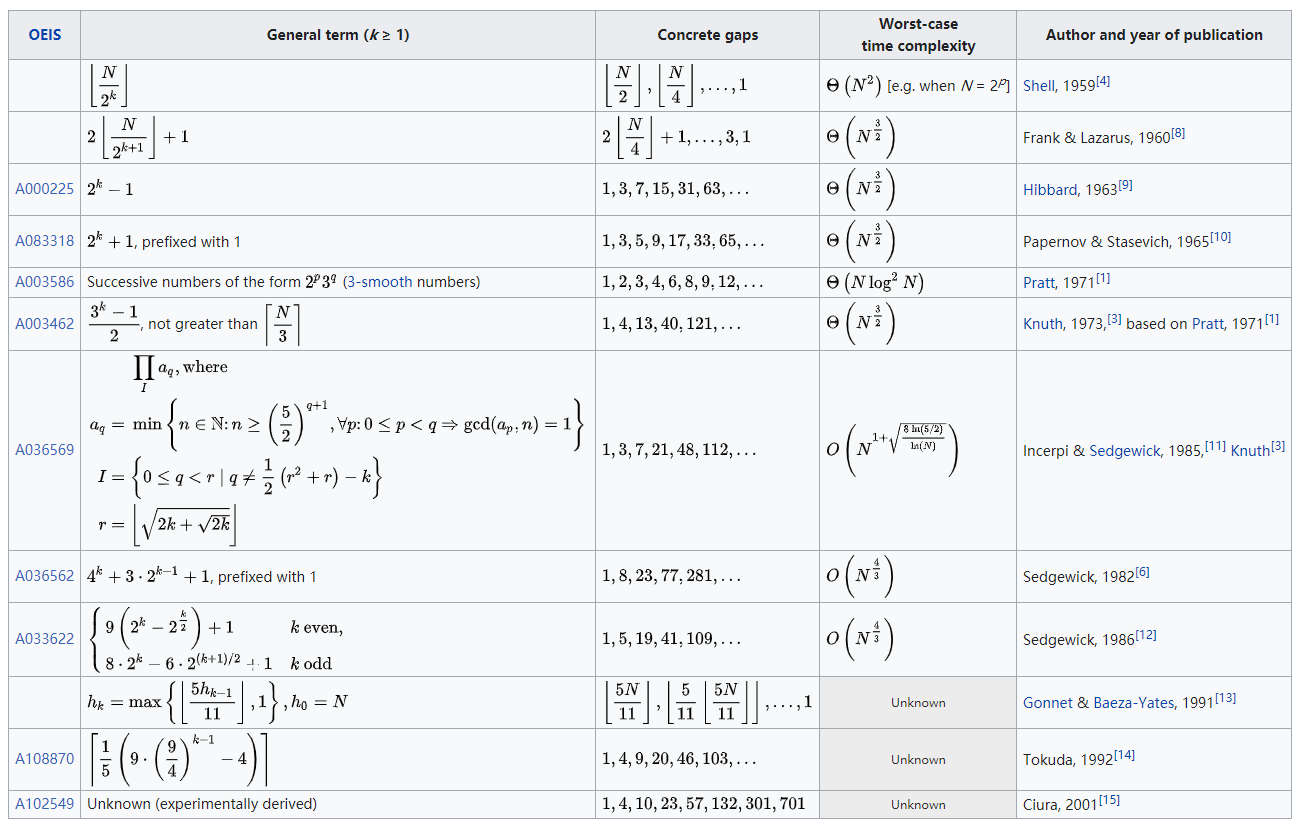# 1 冒泡排序

``````public int[] bubbleSort(int[] array) {
if (array == null || array.length < 2) {
return array;
}
for (int i = 0; i < array.length - 1; i++) {
boolean flag = false;
for (int j = 0; j < array.length - 1 - i; j++) {
if (array[j] > array[j + 1]) {
//这里交换两个数据并没有使用中间变量，而是使用异或的方式来实现
array[j] = array[j] ^ array[j + 1];
array[j + 1] = array[j] ^ array[j + 1];
array[j] = array[j] ^ array[j + 1];

flag = true;
}
}
if (!flag) {
break;
}
}
return array;
}
``````

# 2 选择排序

``````public int[] selectSort(int[] array) {
if (array == null || array.length < 2) {
return array;
}
for (int i = 0; i < array.length; i++) {
int minIndex = i;
for (int j = i + 1; j < array.length; j++) {
if (array[j] < array[minIndex]) {
minIndex = j;
}
}
if (minIndex > i) {
array[i] = array[i] ^ array[minIndex];
array[minIndex] = array[i] ^ array[minIndex];
array[i] = array[i] ^ array[minIndex];
}
}
return array;
}
``````

# 3 插入排序

``````public int[] insertSort(int[] array) {
if (array == null || array.length < 2) {
return array;
}
for (int i = 1; i < array.length; i++) {
int temp = array[i];
int j = i - 1;
while (j >= 0 && array[j] > temp) {
array[j + 1] = array[j];
j--;
}
array[j + 1] = temp;
}
return array;
}
``````

# 4 希尔排序``````public int[] shellSort(int[] array) {
if (array == null || array.length < 2) {
return array;
}
int gap = array.length >>> 1;
while (gap > 0) {
for (int i = gap; i < array.length; i++) {
int temp = array[i];
int j = i - gap;
while (j >= 0 && array[j] > temp) {
array[j + gap] = array[j];
j = j - gap;
}
array[j + gap] = temp;
}
gap >>>= 1;
}
return array;
}
``````

# 5 堆排序

``````public class MaxHeap {

/**
* 排序数组
*/
private int[] nodeArray;
/**
* 数组的真实大小
*/
private int size;

private int parent(int index) {
return (index - 1) >>> 1;
}

private int leftChild(int index) {
return (index << 1) + 1;
}

private int rightChild(int index) {
return (index << 1) + 2;
}

private void swap(int i, int j) {
nodeArray[i] = nodeArray[i] ^ nodeArray[j];
nodeArray[j] = nodeArray[i] ^ nodeArray[j];
nodeArray[i] = nodeArray[i] ^ nodeArray[j];
}

private void siftUp(int index) {
//如果index处节点的值大于其父节点的值，则交换两个节点值，同时将index指向其父节点，继续向上循环判断
while (index > 0 && nodeArray[index] > nodeArray[parent(index)]) {
swap(index, parent(index));
index = parent(index);
}
}

private void siftDown(int index) {
//左孩子的索引比size小，意味着索引index处的节点有左孩子，证明此时index节点不是叶子节点
while (leftChild(index) < size) {
//maxIndex记录的是index节点左右孩子中最大值的索引
int maxIndex = leftChild(index);
//右孩子的索引小于size意味着index节点含有右孩子
if (rightChild(index) < size && nodeArray[rightChild(index)] > nodeArray[maxIndex]) {
maxIndex = rightChild(index);
}
//如果index节点值比左右孩子值都大，则终止循环
if (nodeArray[index] >= nodeArray[maxIndex]) {
break;
}
//否则进行交换，将index指向其交换的左孩子或右孩子，继续向下循环，直到叶子节点
swap(index, maxIndex);
index = maxIndex;
}
}

nodeArray[size] = value;
size++;
//构建大顶堆
siftUp(size - 1);
}

private void extractMax() {
//将堆顶元素和最后一个元素进行交换
swap(0, size - 1);
//此时并没有删除元素，而只是将size-1，剩下的元素重新构建成大顶堆
size--;
//重新构建大顶堆
siftDown(0);
}

public int[] heapSort(int[] array) {
if (array == null || array.length < 2) {
return array;
}
nodeArray = new int[array.length];
for (int value : array) {
}
for (int i = 0; i < array.length; i++) {
extractMax();
}
return nodeArray;
}
}
``````

# 6 归并排序

``````public int[] mergeSort(int[] array) {
if (array == null || array.length < 2) {
return array;
}
return mergeSort(array, 0, array.length - 1);
}

private int[] mergeSort(int[] array, int left, int right) {
if (left < right) {
//这里没有选择“(left + right) / 2”的方式，是为了防止数据溢出
int mid = left + ((right - left) >>> 1);
// 拆分子数组
mergeSort(array, left, mid);
mergeSort(array, mid + 1, right);
// 对子数组进行合并
merge(array, left, mid, right);
}
return array;
}

private void merge(int[] array, int left, int mid, int right) {
int[] temp = new int[right - left + 1];
// p1和p2为需要对比的两个数组的指针，k为存放temp数组的指针
int p1 = left, p2 = mid + 1, k = 0;
while (p1 <= mid && p2 <= right) {
if (array[p1] <= array[p2]) {
temp[k++] = array[p1++];
} else {
temp[k++] = array[p2++];
}
}
// 把剩余的数组直接放到temp数组中
while (p1 <= mid) {
temp[k++] = array[p1++];
}
while (p2 <= right) {
temp[k++] = array[p2++];
}
// 复制回原数组
for (int i = 0; i < temp.length; i++) {
array[i + left] = temp[i];
}
}
``````

# 7 快速排序``````private static final int THRESHOLD = 47;

public int[] quickSort(int[] array) {
if (array == null || array.length < 2) {
return array;
}
return quickSort(array, 0, array.length - 1);
}

private int[] quickSort(int[] array, int start, int end) {
// 如果当前需要排序的数据量小于等于THRESHOLD则走插入排序的逻辑，否则继续走快速排序
if (end - start <= THRESHOLD - 1) {
return insertSort(array);
}

// left和right指针分别指向array的第一个和最后一个元素
int left = start, right = end;

/*
取最左边、最右边和最中间的三个元素的中间值作为基准数据，以此来尽量避免每次都取第一个值作为基准数据、
时间复杂度可能退化为O(n^2)的情况出现
*/
int middleOf3Indexs = middleOf3Indexs(array, start, end);
if (middleOf3Indexs != start) {
swap(array, middleOf3Indexs, start);
}

// temp存放的是array中需要比较的基准数据
int temp = array[start];

while (left < right) {
// 首先从right指针开始比较，如果right指针位置处数据大于temp，则将right指针左移
while (left < right && array[right] >= temp) {
right--;
}
// 如果找到一个right指针位置处数据小于temp，则将right指针处数据赋给left指针处
if (left < right) {
array[left] = array[right];
left++;
}
// 然后从left指针开始比较，如果left指针位置处数据小于temp，则将left指针右移
while (left < right && array[left] <= temp) {
left++;
}
// 如果找到一个left指针位置处数据大于temp，则将left指针处数据赋给right指针处
if (left < right) {
array[right] = array[left];
right--;
}
}
// 当left和right指针相等时，此时循环跳出，将之前存放的基准数据赋给当前两个指针共同指向的数据处
array[left] = temp;
// 一次替换后，递归交换基准数据左边的数据
if (start < left - 1) {
array = quickSort(array, start, left - 1);
}
// 之后递归交换基准数据右边的数据
if (right + 1 < end) {
array = quickSort(array, right + 1, end);
}
return array;
}

private int middleOf3Indexs(int[] array, int start, int end) {
int mid = start + ((end - start) >>> 1);
if (array[start] < array[mid]) {
if (array[mid] < array[end]) {
return mid;
} else {
return array[start] < array[end] ? end : start;
}
} else {
if (array[mid] > array[end]) {
return mid;
} else {
return array[start] < array[end] ? start : end;
}
}
}

private void swap(int[] array, int i, int j) {
array[i] = array[i] ^ array[j];
array[j] = array[i] ^ array[j];
array[i] = array[i] ^ array[j];
}
``````

# 8 计数排序

``````public int[] countingSort(int[] array) {
if (array == null || array.length < 2) {
return array;
}
//记录待排序数组中的最大值
int max = array;
//记录待排序数组中的最小值
int min = array;
for (int i : array) {
if (i > max) {
max = i;
}
if (i < min) {
min = i;
}
}
int[] temp = new int[max - min + 1];
//记录每个数出现的次数
for (int i : array) {
temp[i - min]++;
}
int index = 0;
for (int i = 0; i < temp.length; i++) {
//当输出一个数之后，当前位置的计数就减一，直到减到0为止
while (temp[i]-- > 0) {
array[index++] = i + min;
}
}
return array;
}
``````

``````public int[] stableCountingSort(int[] array) {
if (array == null || array.length < 2) {
return array;
}
//记录待排序数组中的最大值
int max = array;
//记录待排序数组中的最小值
int min = array;
for (int i : array) {
if (i > max) {
max = i;
}
if (i < min) {
min = i;
}
}
int[] temp = new int[max - min + 1];
//记录每个数出现的次数
for (int i : array) {
temp[i - min]++;
}
//将temp数组进行转换，记录每个数在最后排好序的数组中应该要存放的位置+1（如果有重复的就记录最后一个）
for (int j = 1; j < temp.length; j++) {
temp[j] += temp[j - 1];
}
int[] sortedArray = new int[array.length];
//这里必须是从后往前遍历，以此来保证稳定性
for (int i = array.length - 1; i >= 0; i--) {
sortedArray[temp[array[i] - min] - 1] = array[i];
temp[array[i] - min]--;
}
return sortedArray;
}
``````

# 9 桶排序

``````public int[] bucketSort(int[] array) {
if (array == null || array.length < 2) {
return array;
}
//记录待排序数组中的最大值
int max = array;
//记录待排序数组中的最小值
int min = array;
for (int i : array) {
if (i > max) {
max = i;
}
if (i < min) {
min = i;
}
}
//计算桶的数量（可以自定义实现）
int bucketNumber = (max - min) / array.length + 1;
List<Integer>[] buckets = new ArrayList[bucketNumber];
//计算每个桶存数的范围（可以自定义实现或者不用实现）
int bucketRange = (max - min + 1) / bucketNumber;

for (int value : array) {
//计算应该放到哪个桶中（可以自定义实现）
int bucketIndex = (value - min) / (bucketRange + 1);
//延迟初始化
if (buckets[bucketIndex] == null) {
buckets[bucketIndex] = new ArrayList<>();
}
//放入指定的桶
}
int index = 0;
for (List<Integer> bucket : buckets) {
//对每个桶进行内部排序，我这里使用的是快速排序，也可以使用别的排序算法，当然也可以继续递归去做桶排序
quickSort(bucket);
if (bucket == null) {
continue;
}
//将不为null的桶中的数据按顺序写回到array数组中
for (Integer integer : bucket) {
array[index++] = integer;
}
}
return array;
}
``````

# 10 基数排序``````public int[] radixSort(int[] array) {
if (array == null || array.length < 2) {
return array;
}
//记录待排序数组中的最大值
int max = array;
for (int i : array) {
if (i > max) {
max = i;
}
}
//获取最大值的位数
int maxDigits = 0;
while (max != 0) {
max /= 10;
maxDigits++;
}
//用来计数排序的临时数组
int[] temp = new int;
//用来存放每轮排序后的结果
int[] sortedArray = new int[array.length];
for (int d = 1; d <= maxDigits; d++) {
//每次循环开始前都要清空temp数组中的值
replaceArray(temp, null);
//记录每个数出现的次数
for (int a : array) {
temp[getNumberFromDigit(a, d)]++;
}
//将temp数组进行转换，记录每个数在最后排好序的数组中应该要存放的位置+1（如果有重复的就记录最后一个）
for (int j = 1; j < temp.length; j++) {
temp[j] += temp[j - 1];
}
//这里必须是从后往前遍历，以此来保证稳定性
for (int i = array.length - 1; i >= 0; i--) {
int index = getNumberFromDigit(array[i], d);
sortedArray[temp[index] - 1] = array[i];
temp[index]--;
}
//一轮计数排序过后，将这次排好序的结果赋值给原数组
replaceArray(array, sortedArray);
}
return array;
}

private final static int[] sizeTable = {1, 10, 100, 1000, 10000, 100000, 1000000, 10000000, 100000000, 1000000000};

/**
* 获取指定位数上的数字是多少
*/
private int getNumberFromDigit(int number, int digit) {
if (digit < 0) {
return -1;
}
return (number / sizeTable[digit - 1]) % 10;
}

private void replaceArray(int[] originalArray, int[] replaceArray) {
if (replaceArray == null) {
for (int i = 0; i < originalArray.length; i++) {
originalArray[i] = 0;
}
} else {
for (int i = 0; i < originalArray.length; i++) {
originalArray[i] = replaceArray[i];
}
}
}
``````

# 11 复杂度及稳定性

（其中：

1. k表示计数排序中最大值和最小值之间的差值；
2. l表示桶排序中桶的个数；
3. d表示基数排序中最大值的位数，r表示是多少进制；
4. 希尔排序的时间复杂度很大程度上取决于增量gap sequence的选择，不同的增量会有不同的时间复杂度。文中使用的“gap=length/2”和“gap=gap/2”是一种常用的方式，也被称为希尔增量，但其并不是最优的。其实希尔排序增量的选择与证明一直都是个数学难题，而下图列出的是迄今为止大部分的gap sequence选择的方案）# 12 小彩蛋：猴子排序

``````public int[] bogoSort(int[] array) {
if (array == null || array.length < 2) {
return array;
}
Random random = new Random();
while (!inOrder(array)) {
for (int i = 0; i < array.length; i++) {
int swapPosition = random.nextInt(i + 1);
if (swapPosition == i) {
continue;
}
array[i] = array[i] ^ array[swapPosition];
array[swapPosition] = array[i] ^ array[swapPosition];
array[i] = array[i] ^ array[swapPosition];
}
}
return array;
}

private boolean inOrder(int[] array) {
for (int i = 0; i < array.length - 1; i++) {
if (array[i] > array[i + 1]) {
return false;
}
}
return true;
}
``````